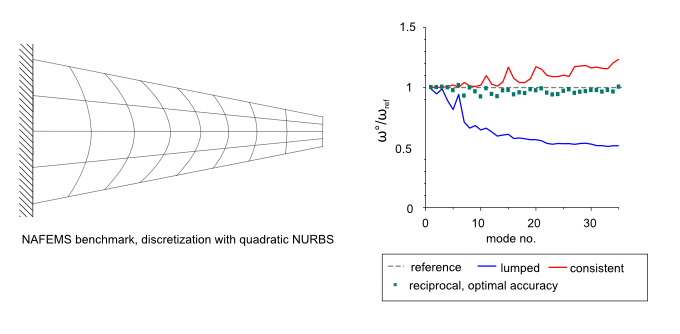# Mass scaling

Completed Research project

Increase of efficiancy and accuracy by variational formulations.

## Overview

• parametric variational principles of dynamics
• increase of efficiency by variationally selective mass and reciprocal mass scaling
• increase of accuracy by variationally constructed inertia templates
• time step estimate for reciprocal mass matrices

## Project description

### Parametric variational principles of dynamics

This research project deals methodically with the further development of parametric variational principles of dynamics. In the preliminary work, a variational formulation based on a modified form of Hamilton’s principle has been introduced. The introduction of several independent fields, i.e. the independent discretizations of the displacement, velocity and linear momentum, allow the derivation of singular mass matrices, and variationally scaled mass and reciprocal mass matrices. While singular mass matrices can be used for the reduction of oscillations in implicit contact problems, the variationally scaled mass and reciprocal mass matrices allow an increase in efficiency in explicit dynamic analyses by increasing the critical time step. A schematic illustration on how selective mass scaling works is given in the figure below.Schematic illustration of the prinicple of selective mass scaling. The maximum eigenfrequency is reduced without influencing significantly the lowest, relevant modes. A reduction of the maximum eigenfrequency results in a larger possible time step.

### Increase of efficiency by variationally selective mass and reciprocal mass scaling

Whereas variational selective mass scaling leads to non-diagonal mass matrices and thus requires the solution of a linear system of equations in each time step of an explicit analysis, the use of reciprocal mass matrices allows a trivial solution to obtain the accelerations. Each time step is therefore similarily expensive as for the commonly used diagonal mass matrix. By the optimal selection of the free parameters in the formulation, an additional increase of the time step by a factor of  2 can be achieved.

While in the initial work, reciprocal mass matrices were only investigated for simplex elements, the extension of the method for a wide range of solid finite elements is carried out and systematically investigated in this project.

In order to make the method usable for practical applications, various routines of the explicit finite element routine must be revised for the use of reciprocal mass matrices. This concerns e.g. the treatment of contact problems or multi-point constraints.

### Increase of accuracy by variationally constructed inertia templates

Alternatively to an increase of efficiency in terms of mass scaling, an increase of accuracy can also be achieved by a systematic tuning of the free parameters in the formulation.

An application example are isogeometric finite elements. For explicit analyses, there are no diagonal mass matrices that provide satisfactory results, especially for higher-order discretizations. By the systematic tuning of the free parameters by means of a dispersion analysis existing higher order mass matrices from literature can be reproduced within a variational framework and aditionally, novel, higher order accurate recirocal mass matrices can be proposed.

In the figure below, the ratio of the scaled eigenfrequency to the reference eigenfrequency is illustrated for the first 30 eigenfrequencies of a NAFEMS benchmark discretized with NURBS. With the optimal reciprocal mass matrix significant smaller error in the eigenfrequencies is observed.Eigenfrequency benchmark. With the reciprocal mass matrix significant higher accuracy can be obtained in the lowest modes than with the lumped or consistent mass matrix.

### Time step estimate for reciprocal mass matrices

In order to exploit the full potential of the new reciprocal mass matrices, the critical time step size need to be efficiently estimated.

Unfortunately, existing elemental time step estimates cannot be used for reciprocal mass matrices, since elemental time step estimates do not necessarily provide conservative results for reciprocal mass matrices. This is due to the non-additivity of reciprocal mass matrices. Therefore, a node-based, local and efficient time step estimate for reciprocal mass matrices based on Gershgorin’s theorem is proposed and analyzed in this project. The influence of the penalty stiffness from penalty contact on the critical time step is as well considered. The results obtained with the proposed time step estimate for a benchmark problem of a highly distorted mesh are illustrated in the figure below.Benchmark problem of a highly distorted mesh with reciprocal mass matrix for testing the novel time step estimate. The contour plot shows the nodal time step, obtained with the novel Gershgorin’s estimate for reciprocal mass matrices.

## Project data

Project titel:
Variational methods for mass scaling
Funding:

German Research Foundation (DFG), Research Grant BI 722/10-1, GEPRIS project number 279006948
Researcher:
Anne-Kathrin Schäuble, Anton Tkachuk

### Publications

1. Schäuble, A.-K. (2019). Variationally conistent inertia templates for speed-up and customization in explicit dynamics. Doktorarbeit, Bericht Nr. 69, Institut für Baustatik und Baudynamik, Universität Stuttgart. https://doi.org/10.18419/opus-10606
2. Schäuble, A.-K., Tkachuk, A., & Bischoff, M. (2018). Time step estimates for explicit dynamics with reciprocal mass matrices. Computers & Structures, 202, 74–84. https://doi.org/10.1016/j.compstruc.2018.03.005
3. Schäuble, A.-K., Tkachuk, A., & Bischoff, M. (2017). Variationally consistent inertia templates for B-spline- and NURBS-based FEM: Inertia scaling and customization. Computer Methods in Applied Mechanics and Engineering, 326, 596–621. https://doi.org/10.1016/j.cma.2017.08.035
4. Tkachuk, A., & Bischoff, M. (2015). Direct and sparse construction of consistent inverse mass matrices: general variational formulation and application to selective mass scaling. International Journal for Numerical Methods in Engineering, 101, 435–469. https://doi.org/10.1002/nme.4805
5. Schäuble, A.-K., Tkachuk, A., & Bischoff, M. (2014). Variationelle Methoden zur Massenskalierung für eine effizientere explizite Zeitintegration in der Dynamik. K.-U. Bletzinger, N. Gebbeken, R. Fisch (eds.): Berichte der Fachtagung Baustatik - Baupraxis 12., 705–713.
6. Tkachuk, A., & Bischoff, M. (2014). Local and global strategies for optimal selective mass scaling. Computational Mechanics, 53, 1197–1207. https://doi.org/10.1007/s00466-013-0961-5
7. Tkachuk, A. (2013). Variational methods for consistent singular and scaled mass matrices. Doktorarbeit, Bericht Nr. 60, Institut für Baustatik und Baudynamik, Universität Stuttgart. https://doi.org/10.18419/opus-517
8. Tkachuk, A., & Bischoff, M. (2013). Variational methods for selective mass scaling. Computational Mechanics, 52, 563–570. https://doi.org/10.1007/s00466-013-0832-0